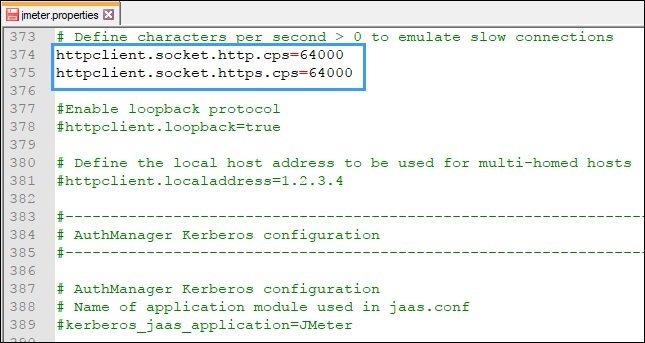# How to simulate network speed in JMeter?

## Steps to simulate the network speed (data transfer speed) in JMeter:

1. Open jmeter.properties file located in /bin folder of JMeter

2. Search for keyword “cps”

3. If you search using “cps” then you will get two properties:

• httpclient.socket.http.cps=0
• httpclient.socket.https.cps=0

Remove “#” to enable the properties

The first property simulates the speed when you use HTTP method (protocol) and the second property is used to simulate the speed for HTTPS method.

4. Calculate the value using below formula or use CPS Calculator:

CPS = RB * 128

where:
CPS = Characters per second
RB = Required Bandwidth that you need to set for the load test. The input value of required bandwidth should be in kbps.Figure 04: Calculate and insert the value for CPS (say 64000)

5. Save and close the file

## Example:

To understand the calculation let’s take an example: If you want to simulate 500 kbps speed for your test then the calculation will be:
=> cps = 500 x 128
=> cps = 64000

So, you need to set:

httpclient.socket.http.cps=64000

Hence JMeter will send the data with the speed of 64000 characters per second and you will achieve 500kbps network bandwidth.

## How to set bandwidth using the command line option (non-GUI mode)?

To set the speed in the non-GUI mode, use the below command:

jmeter -Jhttpclient.socket.http.cps=<calculated value> -n -t <path of .jmx> -l <path of .jtl>

Points to remember:

• Check the testing environment network bandwidth (where the test to be conducted) before simulating the speed. Because JMeter can only simulate the bandwidth equal to or less than the testing environment network bandwidth.
• Use RB (Required Bandwidth) value in kbps for CPS calculation.